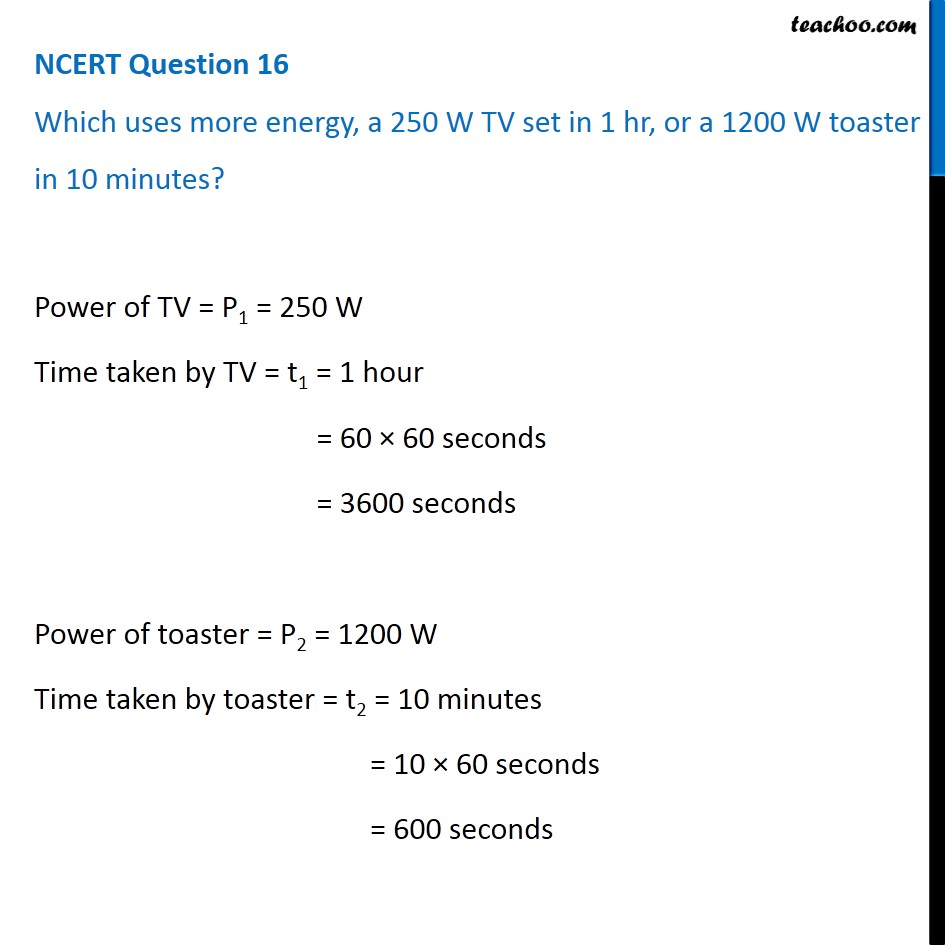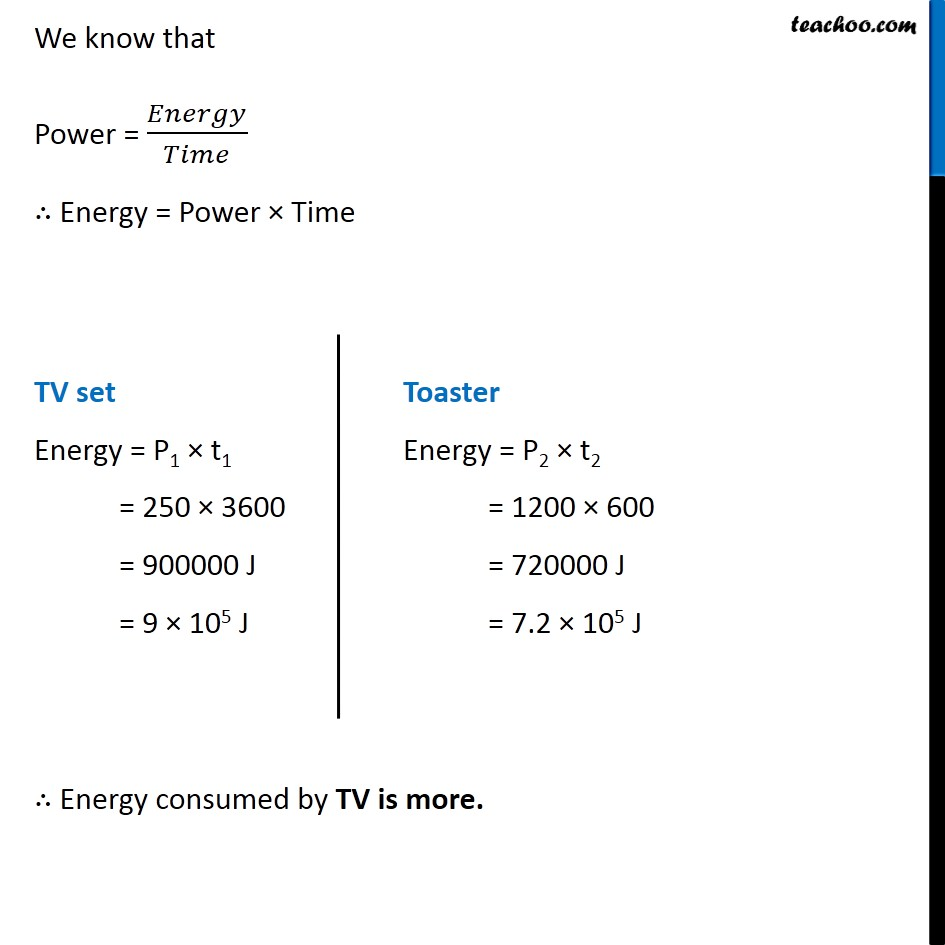NCERT Questions

Class 10
Chapter 12 Class 10 - Electricity

## Which uses more energy, a 250 W TV set in 1 hr, or a 1200 W toaster in 10 minutes?Learn in your speed, with individual attention - Teachoo Maths 1-on-1 Class

### Transcript

NCERT Question 16 Which uses more energy, a 250 W TV set in 1 hr, or a 1200 W toaster in 10 minutes? Power of TV = P1 = 250 W Time taken by TV = t1 = 1 hour = 60 × 60 seconds = 3600 seconds Power of toaster = P2 = 1200 W Time taken by toaster = t2 = 10 minutes = 10 × 60 seconds = 600 seconds We know that Power = 𝐸𝑛𝑒𝑟𝑔𝑦/𝑇𝑖𝑚𝑒 ∴ Energy = Power × Time TV set Energy = P1 × t1 = 250 × 3600 = 900000 J = 9 × 105 J Toaster Energy = P2 × t2 = 1200 × 600 = 720000 J = 7.2 × 105 J ∴ Energy consumed by TV is more.